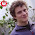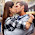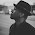## QuickSort Algorithm Tutorial

We have already done tutorial on Merge Sort and a tutorial on Heap Sort (Array Based) with both having a time complexity of O(n*log n). Here is another algorithm which has a time complexity of O(n*log n) and it's called QuickSort.

QuickSort as we all know has a similar approach to Merge Sort i.e. it uses Divide-and-Conquer recursive algorithm to sort the values. The difference being is it's an in-place sorting algorithm.

Basically an in-place algorithm is one which transforms the input using a data structure with a small, constant amount of extra storage space.

# Basic Idea of QuickSort

1. Pick an element in the array as the pivot element.

2. Make a pass to the array, called the PARTITION step, which rearranges the elements in the array:
a. The pivot element is in the proper place
b. The elements less than pivot element are on the left of it
c. The elements greater than pivot element are on the right of it
3. Recursively apply the above process to the left and right part of the pivot element.

# Algorithm

Step 1.  Choosing the Pivot Element
Choosing the pivot element can determine the complexity of the algorithm i.e. whether it will be n*logn or quadratic time:

a. Normally we choose the first, last or the middle element as pivot. This can harm us badly as the pivot might end up to be the smallest or the largest element, thus leaving one of the partitions empty.

b. We should choose the Median of the first, last and middle elements. If there are N elements, then the ceiling of N/2 is taken as the pivot element.

Example:

8, 3, 25, 6, 10, 17, 1, 2, 18, 5

first element: 8
middle element: 10
last element: 5

Therefore the median on [8,10,5] is 8.

Step 2.  Partitioning

a. First thing is to get the pivot out of the way and swapping it with the last number.

Example: (shown using the above array elements)

5, 3, 25, 6, 10, 17, 1, 2, 18,  8

b. Now we want the elements greater than pivot to be on the right side of it and similarly the elements less than pivot to be on the left side of it.

For this we define 2 pointers, namely i and j. i being at the first index and j being and the last index of the array.

* While i is less than j  we keep in incrementing i until we find an element    greater than pivot.
* Similarly, while j is greater then i keep decrementing j  until we find an element less than pivot.
* After both i and j stop we swap the elements at the indexes of i and j respectively.

c. Restoring the pivot

When the above steps are done correctly we will get this as our output:

[5, 3, 2, 6, 1]  [10, 25, 18, 17]

Step 3. Recursively Sort the left and right part of the pivot.

# Java Code

```public class QuickSortAlgorithm {

protected static int A[];

public void sort(int[] A) {
if (A == null || A.length == 0)
return;
quicksort(A, 0, A.length - 1);
}

public void quicksort(int[] A, int left, int right) {
int pivot = A[left + (right - left) / 2];
int i = left;
int j = right;
while (i <= j) {
while (A[i] < pivot) {
i++;
}
while (A[j] > pivot) {
j--;
}
if (i <= j) {
exchange(i, j);
i++;
j--;
}
}

if(left < j)
quicksort(A,left,j);
if(i < right)
quicksort(A,i,right);
}

public void exchange(int i, int j){
int temp=A[i];
A[i]=A[j];
A[j]=temp;
}

public String toString() {
String s = "";
s += "[" + A;
for (int i = 1; i < A.length; i++) {
s += ", " + A[i];
}
s += "]";
return s;
}

}

```

# Complexity of QuickSort

Worst Case : O(N^2)

This happens when the pivot is the smallest or the largest element. Then one of the partition is empty and we repeat the recursion for N-1 elements

Best Case: O(NlogN)

This is when the pivot is the median of the array and the left and right part are the of the same size.

There are logN partitions and to compare we do N comparisions

# Conclusion :

* One of the fastest algorithm on average.
* Doesn't need additional memory i.e. it's an in-place sorting algorithm.

Worst Case complexity is O(N^2).

Comparision to Merge Sort

* Merge Sort guarantee O(NlogN) time, however it requires additional memory with size N.
* Quick Sort doesn't require additional memory but the running time is not guaranteed.
* Usually Merge Sort is not used for Memory Sorting. Its used only for external memory sorting.

Do leave your comments and let me know if I am wrong somewhere. Thanks!

1.Small correction:
public void sort(int[] A) {
if (A == null || A.length == 0)
return;
quicksort(A, 0, A.length - 1);
}

1.Well the same is mentioned on the post!!

2.I made a visualization on the walnut that makes it a bit more clear: https://thewalnut.io/visualizer/visualize/3474/334/

You can also see explain graphically how the choice of pivot affects running time: Compare the call tree at https://thewalnut.io/visualizer/visualize/3474/426/#time=41 vs https://thewalnut.io/visualizer/visualize/3474/426/#time=41

3.The walnut link is not working..

4.Hi,

In part b of step 1, It is mentioned to take median of first, last and middle element.
For taking median, you first need to sort these elements then you can say that the middle element is median.

1.5.Nice article and your are sharing a wonderful information to the readers. The tutorial is very simple to learn and informative. I would like to thank you for sharing this good article.

6.Finance Dissertation Help
I loved the way you discuss the topic great work thanks for the share Your informative post.

7.8.First thing is to get the pivot out of the way and swapping it with the last number.
Are we executing this step in code , I couldn't figure it out.

9.the program is best but it is so complex to understand

10.Thanks for sharing this article. That was very useful. Visit also this source to know how to spy on whatsapp messages.